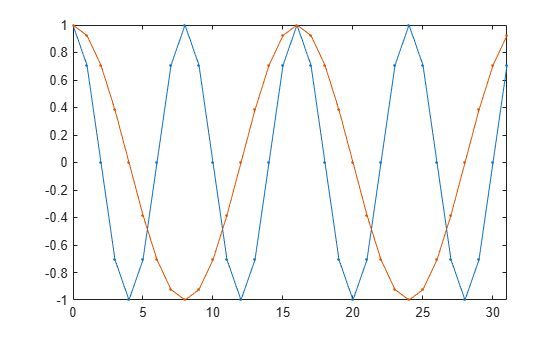# seqperiod

Compute period of sequence

## Syntax

``p = seqperiod(x)``
``p = seqperiod(x,tol)``
``[p,nr] = seqperiod(x)``

## Description

example

````p = seqperiod(x)` returns the integers that correspond to the periods of the sequences in `x`. The period `p` is computed as the minimum length of a subsequence `x(1:p)` of `x` that repeats itself continuously every `p` samples.```

example

````p = seqperiod(x,tol)` specifies `tol` as the absolute tolerance to determine when two numbers are close enough to be treated as equal.```

example

````[p,nr] = seqperiod(x)` also returns the number of repetitions of `x(1:p)` in `x`.```

## Examples

collapse all

Generate a multichannel signal and determine the period of each column.

```x = [4 0 1 6; 2 0 2 7; 4 0 1 5; 2 0 5 6]; p = seqperiod(x)```
```p = 1×4 2 1 4 3 ```

The first column of `x` has period 2. The second column of `x` has period 1. The third column of `x` is not periodic, so `p(3)` is just the number of rows of `x`. The fourth column of `x` has period 3, although the second repetition of the periodic sequence is incomplete.

Compute the number of times that each periodic sequence is repeated.

`[~,nr] = seqperiod(x)`
```nr = 1×4 2.0000 4.0000 1.0000 1.3333 ```

In the first column of `x`, the periodic sequence appears twice. In the second column, the one-sample sequence is repeated as many times as there are samples. In the third column, there is no repetition. The number of repetitions in the fourth column is one plus the fraction of the sequence length represented by the remaining sample.

Generate a two-channel sinusoid such that one channel has four periods in the sampling interval and the other channel has two periods. Plot the sinusoid.

```n = 0:31; x = cos(2*pi./[8;16].*n)'; plot(n,x,'.-') axis tight```Compute the lengths of the repeated subsequences and the number of repetitions. Specify an absolute tolerance of `1e-5`.

`[p,nr] = seqperiod(x,1e-5)`
```p = 1×2 8 16 ```
```nr = 1×2 4 2 ```

Create an array whose first two dimensions have size 1. Along the third dimension, the array has a repeating sequence.

`a = permute([5 4 3 5 4 3 5 4],[3 1 2])`
```a = a(:,:,1) = 5 a(:,:,2) = 4 a(:,:,3) = 3 a(:,:,4) = 5 a(:,:,5) = 4 a(:,:,6) = 3 a(:,:,7) = 5 a(:,:,8) = 4 ```

Compute the period of the repeating sequence and the number of repetitions contained in the array. The function works along the third dimension, as expected.

`[p,nr] = seqperiod(a)`
```p = 3 ```
```nr = 2.6667 ```

## Input Arguments

collapse all

Input array, specified as a vector, matrix, or N-D array.

• If `x` is a matrix, then `seqperiod` checks for periodicity along each column of `x`.

• If `x` is a multidimensional array, then `seqperiod` checks for periodicity along the first array dimension of `x` with size greater than 1.

The length of `x` does not have to be a multiple of `p`, so that incomplete repetitions are permitted at the end of `x`.

Example: `sin(pi./[4;2]*(0:159))'` specifies a two-channel sinusoid. The second channel has twice the frequency of the first channel.

Data Types: `double`

Absolute tolerance to determine when two numbers are close enough to be treated as equal, specified as a positive real scalar.

Data Types: `double`

## Output Arguments

collapse all

Sequence period, returned as a scalar, vector, matrix, or N-D array. If a sequence is not periodic, then `p` equals the length of `x` along the chosen dimension.

• If `x` is a matrix, then `p` is a row vector with the same number of columns as `x`.

• If `x` is a multidimensional array, then `p` is a multidimensional array of integers whose first dimension is of size 1. The remaining dimensions of `p` correspond to the remaining dimensions of `x` with sizes larger than 1.

Number of sequence repetitions, returned as a scalar, vector, matrix, or N-D array. `nr` has the same dimensions as `p`. The elements of `nr` are not necessarily integers.

## Version History

Introduced before R2006a